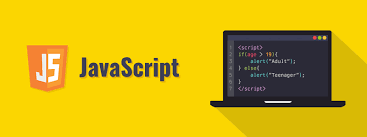# JavaScript For Beginners….

JavaScript is Case sensitive Language and is based on server site and browser. It helps the Programmer to develop the website and maintaining the website. Now I am going to tell you the most important topic of javascript for beginners-

JavaScript value types:Number,String,Boolean,Function,Objectetc.

Number: It has many parts.BigInt,parseInt,parseFloat,Math,NaN etc.

In parseInt, you can convert a string into an int number like =>`parseInt('123', 10);Ans is 123`

You can also convert a floating number using parseFloat() function like=>parseFloat(‘123.5’,10)Ans is 123.5

NaN means not a number. It is returned when the string is non-numeric like =>`parseInt('Hello Tanvir', 10); Ans is NaN;`

The string is called a set of characters. It may be single or multiple characters doesn’t mind. But String is always defined as ‘Hello’ or ‘a’ or ‘ ’it is called null string. It can access with its length. Like => ‘Tanvir’.length ans is 6.

The next One is Boolean. true and false is the main topic of Boolean. It can be logical && and logical ||. False is O, NaN, Undefined, null, empty string “ ” and all others value is true. like => Boolean(‘ ’) Ans is false and Boolean(123)Ans is true

Now we are talking about Function which is very important for beginners because in javascript there are so many functions that can be used. So Function is the most important element of javascript. Example of a function =>

Function sum(a,b)

{

const total=a+b;

return total;

}

Here we can see a Function that has two parameters, A function has 0 parameters or multiple parameters depends on the structure. Then it has the body, In the body, We can give a statement and last of all return the function, if you did not return the function the result will be undefined. So you must have returned the function.

The object is one of the most important elements of javascript.it has a huge field. Array, Function, string one the set of objects.

The array is a special type of object either it is called a set of the same data type. There are many different ways to declare an array.like=>

`const a = new Array();a = 'anamul';a = 'rakib';a = 'jony';`

Another way to declare array=> const a=[‘tanvir’, ‘Rafi, ‘rakib’];

You have must know that the array index starts at 0 and finished incrementally.it means if you have 100 elements then the array index will be 101.Array has so many method like ConCat(),reverse(),Slice(),split(),filter(),find(),forEach(),map(),indexof(),join(),lastindexof(),pop(),push(),shift(),unshift(),reduce(),toString(),sort()etc.

javascript has also Operator and operand.There are some numeric operator.like=> +,-,*,/.it has also reminder operator %.

a+=5;

a=a+5;

++=Increament

— — =Decreament

JavaScript also many conditional statement like if,else,else if=>

if(a>b){console.log(‘a is greater than b’);}

else{(‘b is greater than a’);}

JavaScript has for loop,do-while loop, and while loop. example =>

for(let i=0;i≤5;i++){

let elements=elements[i];

console.log(elements);

}

let i=0;

while(i≤5){let elements=elements[i];

i++;}

I hope it will be helpful for beginners. Thank you for reading.